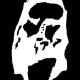# Quod Erat Demonstrandum

## 2014/09/07

### 數算題

Filed under: NSS — johnmayhk @ 12:13 下午
Tags:$nC^{2n-1}_{n-1}=(C^n_1)^2+2(C^n_2)^2+3(C^n_3)^2+\dots +n(C^n_n)^2$$(C^n_1)^2+2(C^n_2)^2+3(C^n_3)^2+\dots +n(C^n_n)^2$$nC^{2n-1}_{n-1}=(C^n_1)^2+2(C^n_2)^2+3(C^n_3)^2+\dots +n(C^n_n)^2$## 2 則迴響 »

1.恆等式左邊的(n-1)和(2n-1)上下倒轉了。

迴響 由 EMK — 2014/09/07 @ 12:20 下午 | 回應

•thx!

迴響 由 johnmayhk — 2014/09/07 @ 12:28 下午 | 回應